# Hidden Markov Models.pptx

28 May 2023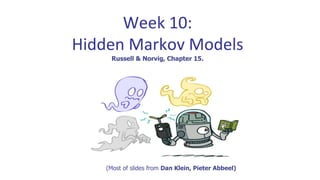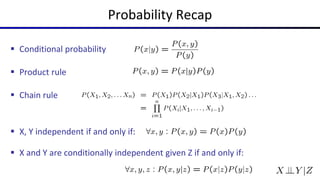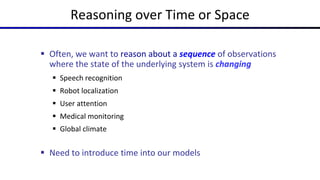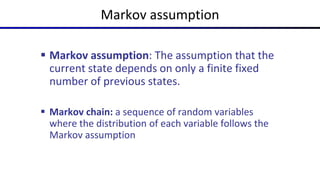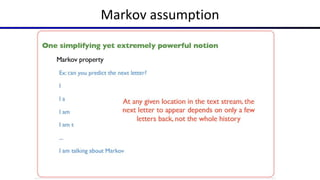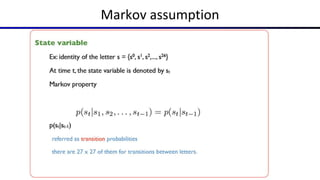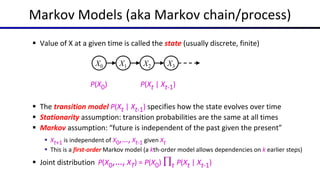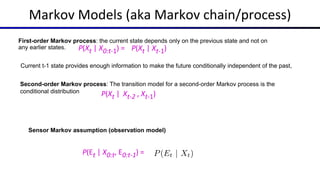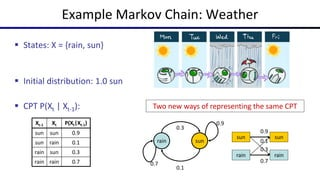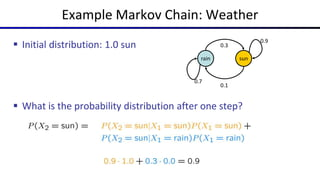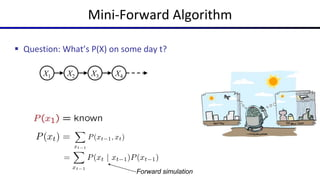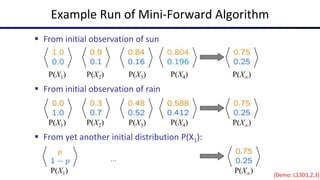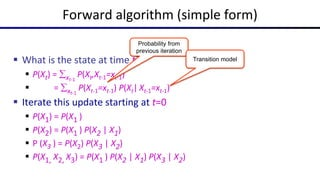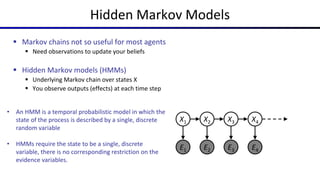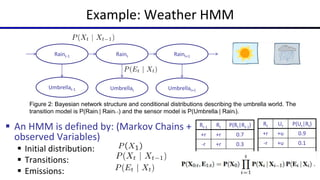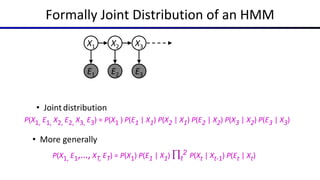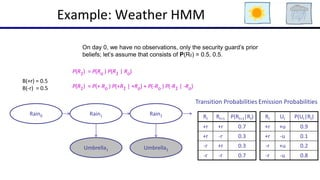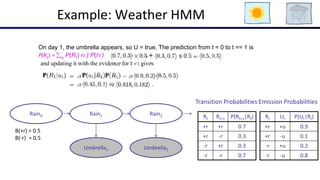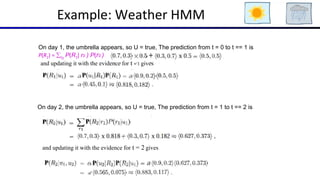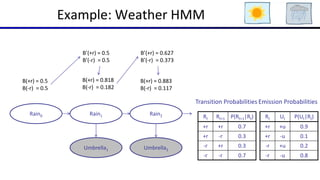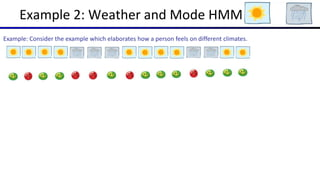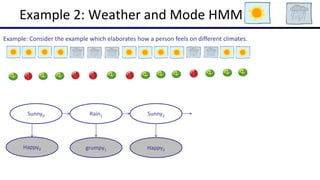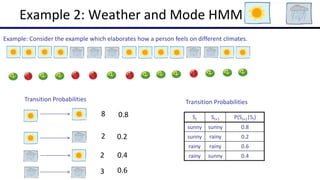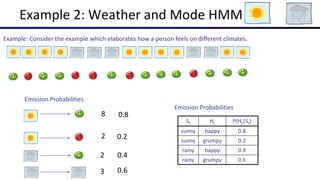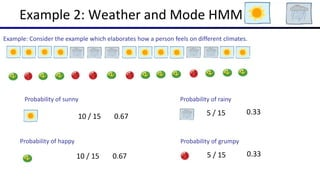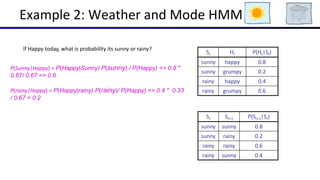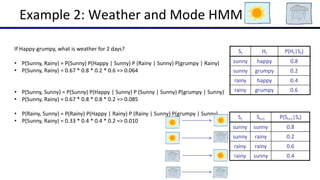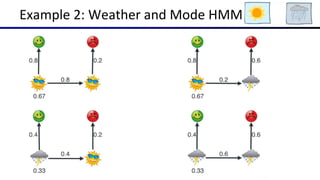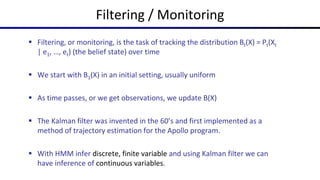1 sur 29

### Hidden Markov Models.pptx

• 1. Week 10: Hidden Markov Models Russell & Norvig, Chapter 15. (Most of slides from Dan Klein, Pieter Abbeel)
• 2. Probability Recap  Conditional probability  Product rule  Chain rule  X, Y independent if and only if:  X and Y are conditionally independent given Z if and only if:
• 3. Reasoning over Time or Space  Often, we want to reason about a sequence of observations where the state of the underlying system is changing  Speech recognition  Robot localization  User attention  Medical monitoring  Global climate  Need to introduce time into our models
• 4. Markov assumption  Markov assumption: The assumption that the current state depends on only a finite fixed number of previous states.  Markov chain: a sequence of random variables where the distribution of each variable follows the Markov assumption
• 7. Markov Models (aka Markov chain/process)  Value of X at a given time is called the state (usually discrete, finite)  The transition model P(Xt | Xt-1) specifies how the state evolves over time  Stationarity assumption: transition probabilities are the same at all times  Markov assumption: “future is independent of the past given the present”  Xt+1 is independent of X0,…, Xt-1 given Xt  This is a first-order Markov model (a kth-order model allows dependencies on k earlier steps)  Joint distribution P(X0,…, XT) = P(X0) t P(Xt | Xt-1) X1 X0 X2 X3 P(X0) P(Xt | Xt-1)
• 8. Markov Models (aka Markov chain/process) P(Xt | Xt-1) First-order Markov process: the current state depends only on the previous state and not on any earlier states. P(Xt | X0:t-1) = Current t-1 state provides enough information to make the future conditionally independent of the past, Second-order Markov process: The transition model for a second-order Markov process is the conditional distribution P(Xt | Xt-2 , Xt-1) Sensor Markov assumption (observation model) P(Et | X0:t, E0:t-1) =
• 9. Example Markov Chain: Weather  States: X = {rain, sun} rain sun 0.9 0.7 0.3 0.1 Two new ways of representing the same CPT sun rain sun rain 0.1 0.9 0.7 0.3 Xt-1 Xt P(Xt|Xt-1) sun sun 0.9 sun rain 0.1 rain sun 0.3 rain rain 0.7  Initial distribution: 1.0 sun  CPT P(Xt | Xt-1):
• 10. Example Markov Chain: Weather  Initial distribution: 1.0 sun  What is the probability distribution after one step? rain sun 0.9 0.7 0.3 0.1
• 11. Mini-Forward Algorithm  Question: What’s P(X) on some day t? Forward simulation X2 X1 X3 X4
• 12. Example Run of Mini-Forward Algorithm  From initial observation of sun  From initial observation of rain  From yet another initial distribution P(X1): P(X1) P(X2) P(X3) P(X) P(X4) P(X1) P(X2) P(X3) P(X) P(X4) P(X1) P(X) … [Demo: L13D1,2,3]
• 13. Forward algorithm (simple form)  What is the state at time t?  P(Xt) = xt-1 P(Xt,Xt-1=xt-1)  = xt-1 P(Xt-1=xt-1) P(Xt| Xt-1=xt-1)  Iterate this update starting at t=0  P(X1) = P(X1 )  P(X2) = P(X1 ) P(X2 | X1)  P (X3 ) = P(X2) P(X3 | X2)  P(X1, X2, X3) = P(X1 ) P(X2 | X1) P(X3 | X2) Probability from previous iteration Transition model
• 14. Hidden Markov Models  Markov chains not so useful for most agents  Need observations to update your beliefs  Hidden Markov models (HMMs)  Underlying Markov chain over states X  You observe outputs (effects) at each time step X5 X2 E1 X1 X3 X4 E2 E3 E4 E5 • An HMM is a temporal probabilistic model in which the state of the process is described by a single, discrete random variable • HMMs require the state to be a single, discrete variable, there is no corresponding restriction on the evidence variables.
• 15. Example: Weather HMM Rt-1 Rt P(Rt|Rt-1) +r +r 0.7 -r +r 0.3 Umbrellat-1 Rt Ut P(Ut|Rt) +r +u 0.9 -r +u 0.1 Umbrellat Umbrellat+1 Raint-1 Raint Raint+1  An HMM is defined by: (Markov Chains + observed Variables)  Initial distribution:  Transitions:  Emissions: Figure 2: Bayesian network structure and conditional distributions describing the umbrella world. The transition model is P(Raint | Raint−1) and the sensor model is P(Umbrellat | Raint).
• 16. Formally Joint Distribution of an HMM X5 E5 X2 E1 X1 X3 E2 E3 P(X1, E1, X2, E2, X3, E3) = P(X1 ) P(E1 | X1) P(X2 | X1) P(E2 | X2) P(X3 | X2) P(E3 | X3) • Jointdistribution P(X1, E1,…, XT, ET) = P(X1) P(E1 | X1) t 2 P(Xt | Xt-1) P(Et | Xt) • More generally
• 17. Example: Weather HMM Rt Rt+1 P(Rt+1|Rt) +r +r 0.7 +r -r 0.3 -r +r 0.3 -r -r 0.7 Rt Ut P(Ut|Rt) +r +u 0.9 +r -u 0.1 -r +u 0.2 -r -u 0.8 Umbrella1 Umbrella2 Rain0 Rain1 Rain2 B(+r) = 0.5 B(-r) = 0.5 On day 0, we have no observations, only the security guard’s prior beliefs; let’s assume that consists of P(R0) = 0.5, 0.5. Transition Probabilities Emission Probabilities P(R1) = P(Ro ) P(R1 | Ro) P(R1) = P(+ Ro ) P(+R1 | +Ro) + P(-Ro ) P(-R1 | -Ro)
• 18. Example: Weather HMM Rt Rt+1 P(Rt+1|Rt) +r +r 0.7 +r -r 0.3 -r +r 0.3 -r -r 0.7 Rt Ut P(Ut|Rt) +r +u 0.9 +r -u 0.1 -r +u 0.2 -r -u 0.8 Umbrella1 Umbrella2 Rain0 Rain1 Rain2 B(+r) = 0.5 B(-r) = 0.5 On day 1, the umbrella appears, so U = true, The prediction from t = 0 to t == 1 is P(R1) = r0 P(R1| r0 ) P(r0 ) and updating it with the evidence for t = 1 gives Transition Probabilities Emission Probabilities
• 19. Example: Weather HMM On day 1, the umbrella appears, so U = true, The prediction from t = 0 to t == 1 is P(R1) = r0 P(R1| r0 ) P(r0 ) and updating it with the evidence for t = 1 gives On day 2, the umbrella appears, so U = true, The prediction from t = 1 to t == 2 is and updating it with the evidence for t = 2 gives
• 20. Example: Weather HMM Rt Rt+1 P(Rt+1|Rt) +r +r 0.7 +r -r 0.3 -r +r 0.3 -r -r 0.7 Rt Ut P(Ut|Rt) +r +u 0.9 +r -u 0.1 -r +u 0.2 -r -u 0.8 Umbrella1 Umbrella2 Rain0 Rain1 Rain2 B(+r) = 0.5 B(-r) = 0.5 B’(+r) = 0.5 B’(-r) = 0.5 B(+r) = 0.818 B(-r) = 0.182 B’(+r) = 0.627 B’(-r) = 0.373 B(+r) = 0.883 B(-r) = 0.117 Emission Probabilities Transition Probabilities
• 21. Example 2: Weather and Mode HMM Example: Consider the example which elaborates how a person feels on different climates.
• 22. Example 2: Weather and Mode HMM grumpy1 Happy2 Sunny0 Rain1 Sunny2 Happy0 Example: Consider the example which elaborates how a person feels on different climates.
• 23. Example 2: Weather and Mode HMM Example: Consider the example which elaborates how a person feels on different climates. Transition Probabilities 8 2 2 3 0.8 0.2 0.4 0.6 St St+1 P(St+1|St) sunny sunny 0.8 sunny rainy 0.2 rainy rainy 0.6 rainy sunny 0.4 Transition Probabilities
• 24. Example 2: Weather and Mode HMM Example: Consider the example which elaborates how a person feels on different climates. Emission Probabilities 8 2 2 3 0.8 0.2 0.4 0.6 St Ht P(Ht|St) sunny happy 0.8 sunny grumpy 0.2 rainy happy 0.4 rainy grumpy 0.6 Emission Probabilities
• 25. Example 2: Weather and Mode HMM Example: Consider the example which elaborates how a person feels on different climates. Probability of sunny 10 / 15 0.67 Probability of rainy 5 / 15 0.33 Probability of happy 10 / 15 0.67 Probability of grumpy 5 / 15 0.33
• 26. Example 2: Weather and Mode HMM St St+1 P(St+1|St) sunny sunny 0.8 sunny rainy 0.2 rainy rainy 0.6 rainy sunny 0.4 St Ht P(Ht|St) sunny happy 0.8 sunny grumpy 0.2 rainy happy 0.4 rainy grumpy 0.6 If Happy today, what is probability its sunny or rainy? P(Sunny|Happy) = P(Happy|Sunny) P(sunny) / P(Happy) => 0.8 * 0.67/ 0.67 => 0.8 P(rainy|Happy) = P(Happy|rainy) P(rainy)/ P(Happy) => 0.4 * 0.33 / 0.67 = 0.2
• 27. Example 2: Weather and Mode HMM St St+1 P(St+1|St) sunny sunny 0.8 sunny rainy 0.2 rainy rainy 0.6 rainy sunny 0.4 St Ht P(Ht|St) sunny happy 0.8 sunny grumpy 0.2 rainy happy 0.4 rainy grumpy 0.6 If Happy-grumpy, what is weather for 2 days? • P(Sunny, Rainy) = P(Sunny) P(Happy | Sunny) P (Rainy | Sunny) P(grumpy | Rainy) • P(Sunny, Rainy) = 0.67 * 0.8 * 0.2 * 0.6 => 0.064 • P(Sunny, Sunny) = P(Sunny) P(Happy | Sunny) P (Sunny | Sunny) P(grumpy | Sunny) • P(Sunny, Rainy) = 0.67 * 0.8 * 0.8 * 0.2 => 0.085 • P(Rainy, Sunny) = P(Rainy) P(Happy | Rainy) P (Rainy | Sunny) P(grumpy | Sunny) • P(Sunny, Rainy) = 0.33 * 0.4 * 0.4 * 0.2 => 0.010
• 28. Example 2: Weather and Mode HMM
• 29. Filtering / Monitoring  Filtering, or monitoring, is the task of tracking the distribution Bt(X) = Pt(Xt | e1, …, et) (the belief state) over time  We start with B1(X) in an initial setting, usually uniform  As time passes, or we get observations, we update B(X)  The Kalman filter was invented in the 60’s and first implemented as a method of trajectory estimation for the Apollo program.  With HMM infer discrete, finite variable and using Kalman filter we can have inference of continuous variables.

### Notes de l'éditeur

1. P(rain) = > P(rain | sun) P(sun) + P (rain|rain) P(rain) 0.1 * 1 + 0.7 * 0 = 0.1
2. P(sun) = > P(sun | sun) P(sun) + P (sun | rain) P(rain) 0.9 * 0.9 + 0.3 * 0.1 = 0.84 P(rain) = > P(rain | sun) P(sun) + P (rain | rain) P(rain) 0.1 * 0.9 + 0.7 * 0.1 = 0.09 + 0.07 = 0.16
3. demo
4. demo
5. The formula for normalization is P (Sunny, Cool) / P (Sunny, Cool) + P (rain, Cool) 0.45 / 0.45 + 0.1  = 0.45/ 0.55  = 0.818 0.1/ 0.55 = 0.1818  P(rain1) = > P(rain1 | rain0) P(rain0) + P (rain1 | - rain0) P(- rain0) P(rain1) = 0.7 * 0.5 + 0.3 * 0.5 = 0.5
6. P (R1 | u1) = P(u1 | R1) P (R1) / P (u1) Remove P (u1) for division to get approximation a = show approximation P (R1 | u1) = a P(u1 | R1) P (R1) P (R1 | u1) = 0.45 P(u1) = 0.55 P (R1 | u1) = 0.45 / 1.1 Procedure: Step 1: Compute Z = sum over all entries Step 2: Divide every entry by Z
7. demo
8. demo
9. demo
10. demo
11. demo
12. demo
13. a = show approximation P(Sunny|Happy) = a P(Happy|Sunny) P(sunny) = a 0.8 * 0.67 = 0.536 P (rainy | happy) = a P(Happy|rainy) P(rainy) = a 0.4 * 0.33 = 0.132 So after approximation ~~ P(Sunny|Happy) = <0.546> = <0.8>
14. demo
15. demo